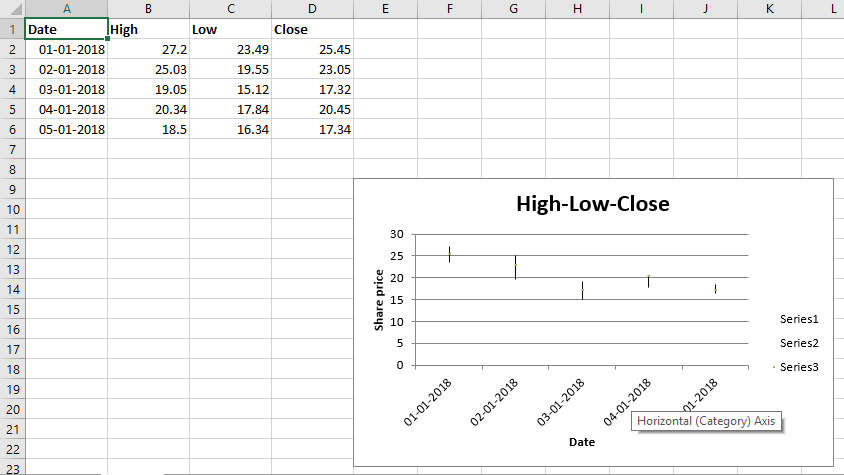# Python | Plotting Stock charts in excel sheet using XlsxWriter module

• Difficulty Level : Medium
• Last Updated : 16 Feb, 2022

Prerequisite: Create and Write on an excel sheet

XlsxWriter is a Python library using which one can perform multiple operations on excel files like creating, writing, arithmetic operations and plotting graphs. Let’s see how to plot Stock charts using realtime data.
Charts are composed of at least one series of one or more data points. Series themselves are comprised of references to cell ranges. For plotting the charts on an excel sheet, firstly, create chart object of specific chart type( i.e Stock chart etc.). After creating chart objects, insert data in it and lastly, add that chart object in the sheet object.

Code : Plot the Stock Chart.

For plotting the Stock chart on an excel sheet, use add_chart() method with type ‘stock’ keyword argument of a workbook object.

## Python3

 `# from datetime library import datetime method``from` `datetime ``import` `datetime` `# import xlsxwriter library``import` `xlsxwriter` `# Workbook() takes one, non-optional, argument  ``# which is the filename that we want to create.``workbook ``=` `xlsxwriter.Workbook(``'chart_stock.xlsx'``)` `# The workbook object is then used to add new  ``# worksheet via the add_worksheet() method. ``worksheet ``=` `workbook.add_worksheet()` `# Create a new Format object to formats cells``# in worksheets using add_format() method .``  ` `# here we create bold format object .``bold ``=` `workbook.add_format({``'bold'``: ``1``})` `# here we create date format object``date_format ``=` `workbook.add_format({``'num_format'``: ``'dd/mm/yyyy'``})` `# Create a chart object that can be added``# to a worksheet using add_chart() method.` `# here we create a stock chart object .``chart ``=` `workbook.add_chart({``'type'``: ``'stock'``})` `# Add the worksheet data that the charts will refer to.``headings ``=` `[``'Date'``, ``'High'``, ``'Low'``, ``'Close'``]``data ``=` `[``    ``[``'2018-01-01'``, ``'2018-01-02'``, ``'2018-01-03'``, ``'2018-01-04'``, ``'2018-01-05'``],``    ``[``27.2``, ``25.03``, ``19.05``, ``20.34``, ``18.5``],``    ``[``23.49``, ``19.55``, ``15.12``, ``17.84``, ``16.34``],``    ``[``25.45``, ``23.05``, ``17.32``, ``20.45``, ``17.34``],``]` `# Write a row of data starting from 'A1'``# with bold format .``worksheet.write_row(``'A1'``, headings, bold)` `# Filling the data in an excel sheet``for` `row ``in` `range``(``5``):` `    ``# convert a string type date into date of respective format.``    ``date ``=` `datetime.strptime(data[``0``][row], ``"% Y-% m-% d"``)` `    ``# apply a date_format object on this particular cell``    ``worksheet.write(row ``+` `1``, ``0``, date, date_format)` `    ``# write data in the respective cells``    ``worksheet.write(row ``+` `1``, ``1``, data[``1``][row])``    ``worksheet.write(row ``+` `1``, ``2``, data[``2``][row])``    ``worksheet.write(row ``+` `1``, ``3``, data[``3``][row])` `# width of A to D column set to 11.``worksheet.set_column(``'A:D'``, ``11``)` `# Add a series for each of the High-Low-Close columns.``# Add a data series to a chart``# using add_series method.` `# note : spaces is not inserted in b / w``# = and Sheet1, Sheet1 and !``# if space is inserted it throws warning.` `# data take from A2 to A6 and B2 to B6 respectively.``chart.add_series({``    ``'categories'``: ``'= Sheet1 !\$A\$2:\$A\$6'``,``    ``'values'``: ``'= Sheet1 !\$B\$2:\$B\$6'``,``})` `# data take from A2 to A6 and C2 to C6 respectively.``chart.add_series({``    ``'categories'``: ``'= Sheet1 !\$A\$2:\$A\$6'``,``    ``'values'``:     ``'= Sheet1 !\$C\$2:\$C\$6'``,``})` `# data take from A2 to A6 and D2 to D6 respectively.``chart.add_series({``    ``'categories'``: ``'= Sheet1 !\$A\$2:\$A\$6'``,``    ``'values'``: ``'= Sheet1 !\$D\$2:\$D\$6'``,``})`  `# Add a chart title ``chart.set_title ({``'name'``: ``'High-Low-Close'``})` `# Add x-axis label``chart.set_x_axis({``'name'``: ``'Date'``})` `# Add y-axis label``chart.set_y_axis({``'name'``: ``'Share price'``})` `# add chart to the worksheet with given``# offset values at the top-left corner of``# a chart is anchored to cell E9``worksheet.insert_chart(``'E9'``, chart)` `# Finally, close the Excel file ``# via the close() method. ``workbook.close()`

Output:My Personal Notes arrow_drop_up